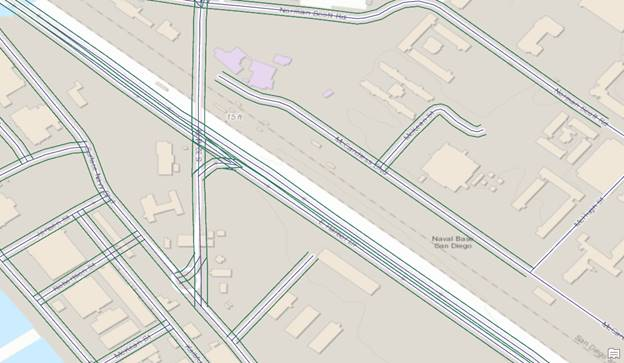English
Continue in the app
Be The First To Get Support Updates

# How To: Create parallel features similar to the Copy Parallel tool in ArcGIS Pro with Python

## Summary

Although ArcGIS Pro has the Create Parallel tool, the tool is not available through ArcPy. As an alternative, a Python script can be used to create a similar effect as seen in the image below. A script may be useful and efficient for workflows related to pipelines, roads and highways.This article provides steps to use a Python script that draws parallel features.

## Procedure

```Note:
This script does not work on line intersections.```
1. Create a clone of the line feature. Refer to ArcGIS Pro: Copy and paste features using the clipboard or ArcGIS Pro: Copy Features (Data Management) for more information.
2. Add a Width field to the clone line feature.
1. Use Add Field in the Fields view of the clone line feature. Refer to ArcGIS Pro: Fields view for more information.
2. Name the field Width and set any numeric data type (long, short) as the data type.
3. Populate the Width field with a value to determine the width of the parallel line from the line feature.
3. Open a Python console or the Python window in ArcGIS Pro.
4. Import the necessary modules.
`import os, arcpy, math`
1. Specify the workspace.
```infc=r'<Feature/Shapefile_Path>'
workspace = os.path.dirname(infc)
edit = arcpy.da.Editor(workspace)```
1. Start editing on the feature.
```edit.startEditing(False,True)
edit.startOperation()```
1. Define a new function to create the parallel line.
```def CopyParallel(plyP,sLength):
part=plyP.getPart(0)
lArray=arcpy.Array()
rArray=arcpy.Array()
for ptX in part:
dL=plyP.measureOnLine(ptX)
ptX0=plyP.positionAlongLine (dL-0.01).firstPoint
ptX1=plyP.positionAlongLine (dL+0.01).firstPoint
dX=float(ptX1.X)-float(ptX0.X)
dY=float(ptX1.Y)-float(ptX0.Y)
lenV=math.hypot(dX,dY)
sX=-dY*sLength/lenV
sY=dX*sLength/lenV
leftP=arcpy.Point(ptX.X+sX,ptX.Y+sY)
rightP=arcpy.Point(ptX.X-sX, ptX.Y-sY)
array = arcpy.Array([lArray, rArray])
section=arcpy.Polyline(array)
return section```
1. Start a new cursor to update the clone line feature to become the parallel line.
```with arcpy.da.UpdateCursor(infc,("Shape@","Width")) as cursor:
for shp,w in cursor:
twoLines=CopyParallel(shp,w)
cursor.updateRow((twoLines,w))```
1. Delete the cursor and stop editing to release the lock file on the feature.
```del cursor
edit.stopOperation()
edit.stopEditing(True)```

The following shows the full script:

```import os, arcpy, math
infc=r'C:\Users\User\Desktop\Work\Sample\Test_Shapefile.shp'
workspace = os.path.dirname(infc)
edit = arcpy.da.Editor(workspace)

edit.startEditing(False,True)
edit.startOperation()

def CopyParallel(plyP,sLength):
part=plyP.getPart(0)
lArray=arcpy.Array()
rArray=arcpy.Array()
for ptX in part:
dL=plyP.measureOnLine(ptX)
ptX0=plyP.positionAlongLine (dL-0.01).firstPoint
ptX1=plyP.positionAlongLine (dL+0.01).firstPoint
dX=float(ptX1.X)-float(ptX0.X)
dY=float(ptX1.Y)-float(ptX0.Y)
lenV=math.hypot(dX,dY)
sX=-dY*sLength/lenV
sY=dX*sLength/lenV
leftP=arcpy.Point(ptX.X+sX,ptX.Y+sY)
rightP=arcpy.Point(ptX.X-sX, ptX.Y-sY)
array = arcpy.Array([lArray, rArray])
section=arcpy.Polyline(array)
return section

with arcpy.da.UpdateCursor(infc,("Shape@","Width")) as cursor:
for shp,w in cursor:
twoLines=CopyParallel(shp,w)
cursor.updateRow((twoLines,w))

del cursor
edit.stopOperation()
edit.stopEditing(True)```

## Related Information

Last Published: 10/15/2020

Article ID: 000024330

Software: ArcGIS Pro 2.6.2, 2.6.1, 2.6, 2.5.2, 2.5.1, 2.5, 2.4.3, 2.4.2, 2.4.1, 2.4, 2.3.3, 2.3.2, 2.3.1, 2.3, 2.2.4, 2.2.3, 2.2.2, 2.2.1, 2.2